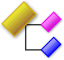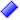# Infinite Impulse Response (namespace IIR)BiquadFilter: It provides support to implement an infinite impulse response filterBiquadFilter(void)bool CreateHighPass(double cutFreq_rads, double passBand_dB, double stopFreq_rads, double stopBand_dB)bool CreateLowPass(double cutFreq_rads, double passBand_dB, double stopFreq_rads, double stopBand_dB)~ BiquadFilter(void)BiquadSection: H(z) = (b0 + b1 * z^-1 + b2 * z ^-2) / (1 + a1 * z^-1 + a2 * z ^-2)BiquadSection()BiquadSection(const IIR::BiquadSection& init)BiquadSection(const IIR::PolarBiquadSection& init)IIR::BiquadSection& operator =(const IIR::BiquadSection& init)IIR::BiquadSection& operator =(const IIR::PolarBiquadSection& init)inline double ComputeOutput(double x)It returns y[n] given a value of x[n] w[n] = x[n] - a1*w[n-1] - a2*w[n-2] y[n] = b0*w[n] + b1*w[n-1] + b2*w[n-2]void Clear()void ComputeOutput(double* buffer, const int numSamples)It modifies the samples in buffer, the operation is in place (that is the output is written in the same buffer)void ComputeOutput_2Channels(double* buffer, const int numSamples)It modifies the samples in buffer, the operation is in place (that is the output is written in the same buffer) buffer has two channels, i.e., ch1, ch2, ch1, ch2, ch1, ch2 (numSamples is 3)void CreateLinkwitzRileyHighPass(double cutFreq_Hertz, double samplingFreq_Hertz)void CreateLinkwitzRileyLowPass(double cutFreq_Hertz, double samplingFreq_Hertz)void ResetTaps()~ BiquadSection()BiquadsCascadeBiquadsCascade()BiquadsCascade(const IIR::BiquadsCascade& init)BiquadsCascade(const IIR::PolarBiquadsCascade& init)IIR::BiquadSection& operator [ ](long index)IIR::BiquadsCascade& operator =(const IIR::BiquadsCascade& init)IIR::BiquadsCascade& operator =(const IIR::PolarBiquadsCascade& init)bool SetSize(int numberBiquadSections)const IIR::BiquadSection& operator [ ](long index)constdouble ComputeOutput(double x)It returns y[n] given a value of x[n]int GetSize()void ComputeOutput(double* buffer, const int numSamples)It modifies the samples in buffer, the operation is in place (that is the output is written in the same buffer)void ComputeOutput_2Channels(double* buffer, const int numSamples)It modifies the samples in buffer, the operation is in place (that is the output is written in the same buffer) buffer has two channels, i.e., ch1, ch2, ch1, ch2, ch1, ch2 (numSamples is 3)void Delete()~ BiquadsCascade()ButterworthFilterButterworthFilter()~ ButterworthFilter()ChebyshevFilterChebyshevFilter()~ ChebyshevFilter()DcRemoverDcRemover()int ComputeOutput(int input)~ DcRemover()EllipticFilterEllipticFilter()~ EllipticFilter()PolarBiquadSection: Andrew G. Deczky 1972 Eq. 10 k (z - zeroR * exp(j * zeroAngle)) (z - zeroR * exp(-j * zeroAngle) ) / (z - poleR *exp (j * poleAngle) ) (z - poleR *exp (-j * poleAngle) )IIR::PolarBiquadSection& operator =(const IIR::BiquadSection& init)IIR::PolarBiquadSection& operator =(const IIR::PolarBiquadSection& init)PolarBiquadSection()PolarBiquadSection(const IIR::BiquadSection& init)PolarBiquadSection(const IIR::PolarBiquadSection& init)void Clear()void GetPoles(double& out_reP1, double& out_imP1, double& out_reP2, double& out_imP2)void GetZeros(double& out_reZ1, double& out_imZ1, double& out_reZ2, double& out_imZ2)~ PolarBiquadSection()PolarBiquadsCascadeIIR::PolarBiquadSection& operator [ ](long index)IIR::PolarBiquadsCascade& operator =(const IIR::BiquadsCascade& init)IIR::PolarBiquadsCascade& operator =(const IIR::PolarBiquadsCascade& init)PolarBiquadsCascade()PolarBiquadsCascade(const IIR::BiquadsCascade& init)PolarBiquadsCascade(const IIR::PolarBiquadsCascade& init)bool SetSize(int numberBiquadSections)const IIR::PolarBiquadSection& operator [ ](long index)constdouble GetGroupDelay(double freq_rads)double GetMagnitude(double freq_rads)int GetSize() constvoid Delete()void GetPoles(vector>& out_poles)void GetZeros(vector>& out_zeros)~ PolarBiquadsCascade()